# Machines

 Pulleys Levers Car hydraulic jack Cranes Cars Our bodies Water wheel Aircraft Bicycle Screw drivers Wind generator Rockets Screw jack CD player Motors Ships

These are all examples of machines that we might meet in the world around us.

A machine is something that can do work, and it usually makes the work easier.
It doesn’t reduce the amount of work done - just the effort needed to do it.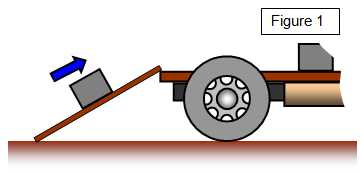An example of a simple machine is a ramp - if you have a heavy box that you want to put on the back of a lorry it is much easier to push it up a ramp than to lift it straight up.

Now the potential energy gained by the box depends only on the vertical height it has moved, and if there is no friction on the ramp the work done will be the same whether the box is pushed up the ramp or lifted straight up. However since the ramp is longer we have to move the effort further and as the work is the same the force needed will be less up the ramp.

Example problem
A 75 kg box has to be lifted 1.5 m onto the back of a lorry, either straight up or by using a 5 m long ramp.
(a) straight lift
work done = 75x10x1.5 = 1125 J

(b) using a 5m ramp
work done = 1125 = 5 x effort
Therefore the effort needed this time = 1125/5 = 225N
So the effort needed in a straight lift is 750 N but only 225 N if we use the ramp.

We call the force that you put into a machine the EFFORT and the force that you move the
LOAD. There are a number of important definitions that are used in connection with machines.

Input work = effort x distance effort moves
Output work = Load x distance load moves
Velocity ratio (VR) = distance effort moves/ distance load moves in the same time### schoolphysics Reciprocating engine animation

To see an animation of a reciprocating engine click on the animation link.

## Efficiency

Now no machine is perfectly efficient – in other words you don't get as much energy out of the machine as you put in and certainly not more. So we define efficiency as:

Efficiency = [energy output/energy input] x 100%

For example if you get out half the energy that you put in, the machine is 50% efficient.
A petrol engine is about 30% efficient, a diesel engine 40% efficient, and our bodies are 25% efficient - only one quarter of the energy produced goes to moving the muscles.

You can also show that:

Efficiency = [mechanical advantage/velocity ratio] x 100%

What happens to all this wasted energy? It is usually lost by friction and this produces heat. Heat is often the waste product of a machine.
We are now going to look at some simple machines. To make things easier we will assume that they are all perfectly efficient.

## The ramp or inclined planeIt is thought that the Ancient Egyptians may have used ramps made of earth or sand to raise the huge stones needed when building the pyramids.

The shallower the ramp the less effort but the further the effort has to move.

Velocity Ratio = length of ramp/height of ramp
Work input = effort x ramp length
Work output = load x height raised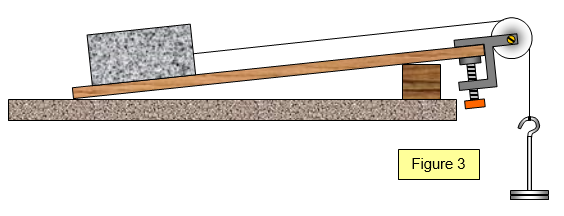## The lever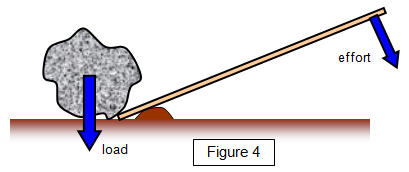Mechanical Advantage = load/effort
Velocity ratio = distance of the effort to the pivot/distance of the load to the pivot

The longer the effort lever the less effort is needed to do a certain job.

Applications: moving rocks, prizing lids of tins, tightening wheel nuts, pliers, nut crackers, tweezers## The wheel and axle

The effort is applied to the larger radius wheel and the load is lifted by the smaller radius axle.

Velocity ratio = diameter of wheel/diameter of axle

Applications: windlass, brace, screwdriver, box spanner, steering wheel.

## Gears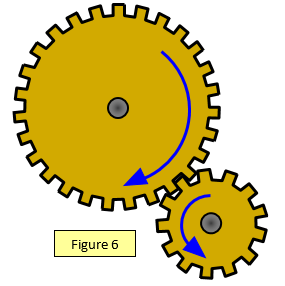Gears are a very widely used application of the wheel and axle. If the load and effort are applied to the two axles then:

Velocity ratio = number of teeth on driven wheel/number of teeth on driving wheel

In the example drawn this is just over 2.

## The screw jack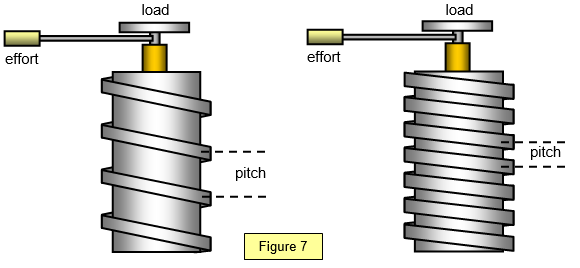When the effort arm makes one complete turn the load is raised by a distance equal to the pitch of the screw.

Velocity Ratio = distance round effort arm circle/ pitch of the screw

## The hydraulic jackThe effort is transmitted to the load through the oil which is taken to be incompressible.